# WiFi信号传播与无线AP定位研究Study of WiFi Signal Propagation and the Location of the Wireless AP

DOI: 10.12677/HJWC.2021.112004, PDF, HTML, XML, 下载: 38  浏览: 125  国家自然科学基金支持

Abstract: Due to its ubiquity, high transmission rate, and free of charge, WiFi is the most popular way to access the Internet, and wireless AP is currently the world’s largest number of radio transceiver devices in use. Therefore, studying WiFi signal propagation and wireless AP positioning is an ideal platform for mastering the basics of radio monitoring technology. Based on the electromagnetic field simulation software WinProp and artificial intelligence algorithm, combined with the radio propagation characteristics of a one-story villa, the wireless AP positioning technology is studied in this paper. The simulation results show that: using convolutional neural network, K neighboring algorithm and support vector machine, when the number of monitoring receiving points is 3, the accuracy rates of identifying wireless AP are 76.7%, 76.3% and 70.0% respectively; when the number is 5, the recognition accuracy rates are 83.6%, 91.4%, and 87.9%, respectively; when the number is 7, the accuracy rates are 92.6%, 98.1%, and 96.8%, respectively. The above work is of great significance for understanding the principle of radio monitoring and positioning in a Non Line of Sight environment.

1. 引言

2. 仿真模型Figure 1. Schematic graph of the appearance of a one-story villa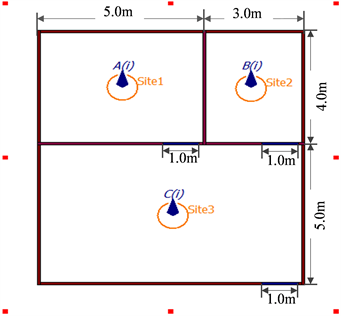Figure 2. Floor plan of wireless AP and receiving point

3. WiFi信号传播特性仿真和数据预处理

WiFi信号传播特性仿真采用WinProp软件 ，仿真时假设无线AP在室内房间内按照从左到右，从上到下的路径共移动5001次，每次移动时进行场分布计算，并在监测接收点接收和记录WiFi信号场强，针对每个房间的仿真将获得8 × 5001组场强数据，为进行发射源定位准备数据。图3(a)-(c)分别是在3个房间发射WiFi信号时得到的仿真区域电场强度分布，仿真时截取的平面高度为1.5 m；图3(d)是在截取高度为1.5 m、2 m和2.5 m的平面上得到的场强分布仿真结果。可以看出，信号最强区域位于无线AP所属房间，靠近该房间的监测接收点测量到的场强次之；当无线AP在房间1时，位于左上角的监测接收点接收到的场强较强，而距离其较远的右下角的监测接收点较弱；同理，当无线AP在房间2和3发射时，位于右上侧和下方的监测接收点接收到的场强较强；当无线AP在各个房间内移动时，随着位置的变化各个监测接收点收到的信号将发生变化。因此，基于接收场强对非视通环境下的无线AP进行定位是可能的。假设每个房间内的无线AP在5001个不同的位置处进行发射，房子外围设置8个监测接收点进行接收时获得的接收功率数据矩阵为：Figure 3. The power distribution inside and outside the villa and at different heights when WiFi signals are transmitted in 3 rooms respectively. (a) Wireless AP is located in room 1; (b) Wireless AP is located in room 2; (c) Wireless AP is located in room 3; (d) The distribution of received power inside and outside the villa under different receiving antenna heights

${P}_{k}=\left[\begin{array}{cccc}{p}_{1,1}& {p}_{1,2}& \cdots & {p}_{1,8}\\ {p}_{2,1}& {p}_{2,2}& \cdots & {p}_{2,8}\\ ⋮& ⋮& \cdots & ⋮\\ {p}_{5001,1}& {p}_{5001,2}& \cdots & {p}_{5001,8}\end{array}\right],k=1,2,3$ (1)

4. 基于AI的发射源定位研究

1) 人工智能AI

SVM是一种监督机器学习算法，本文采用成对分类方法将非线性多分类问题转为binary SVM进行处理。设给定含N个样本的训练数据集 $X=\left\{\left({x}_{1},{y}_{1}\right),\left({x}_{1},{y}_{1}\right),\cdots ,\left({x}_{n},{y}_{n}\right)\right\}$，对于第i类和第j类数据，训练一个SVM分类模型即等效为求解二次规划问题：

$\begin{array}{l}{\mathrm{min}}_{{w}^{ij},{b}^{ij},{\xi }^{ij}}\frac{1}{2}{\left({w}^{ij}\right)}^{T}{w}^{ij}+C\underset{t}{\sum }{\xi }_{t}^{ij}\\ \text{s}\text{.t}\text{.}{\left({w}^{ij}\right)}^{T}\varphi \left({x}_{t}\right)+{b}^{ij}\ge 1-{\xi }_{t}^{ij},\text{if}{y}_{t}=i\\ {\left({w}^{ij}\right)}^{T}\varphi \left({x}_{t}\right)+{b}^{ij}\le -1+{\xi }_{t}^{ij},\text{if}{y}_{t}=j\\ {\xi }_{t}^{ij}\ge 0\end{array}$ (2)

$\varphi \left({x}_{i},{y}_{i}\right)={\text{e}}^{-\gamma ||{x}_{i}-{{y}_{i}|}^{2}},\gamma >0$ (3)

${y}_{\text{new}}^{ij}=\mathrm{sign}\left[{\left({w}^{ij}\right)}^{T}\varphi \left({x}_{new}\right)+{b}^{ij}\right]=\mathrm{sign}\left[\underset{\begin{array}{l}\text{suppor}t\\ \text{vecters}\end{array}}{\sum }{y}_{t}{\alpha }_{i}^{ij}k\left({x}_{t},{x}_{new}\right)+{b}^{ij}\right]$ (4)

(4)式用于判断数据是属于i类还是j类。

KNN是数据挖掘分类技术中最简单的机器学习算法之一，其指导思想是“近朱者赤，近墨者黑”，即由你的邻居来推断出你的类别。KNN算法的核心思想是：为了判断未知样本的类别，以所有已知类别的样本作为参照，计算未知样本与所有已知样本之间的欧式距离，从中选取与未知样本距离最近的K个已知样本，根据少数服从多数的投票法则，将未知样本与K个最邻近样本中所属类别占比较多的归为一类。假设给定两个样本 $X=\left({x}_{1},{x}_{2},\cdots ,{x}_{n}\right)$$Y=\left({y}_{1},{y}_{2},\cdots ,{y}_{n}\right)$，其中n表示特征数，X和Y两个向量间的欧氏距离表示为：

${\mathrm{dist}}_{ed}\left(X,Y\right)=\sqrt{{\left({x}_{1}-{y}_{1}\right)}^{2}+\cdots +{\left({x}_{n}-{y}_{n}\right)}^{2}}$ (5)

KNN算法的分类原理如图4所示，图中当选择的K值取3时，待测样本的预测标签为类别1；当K取5时，待测样本预测标签为类别2。因此K值的选取十分重要，K越小越容易过拟合，当K = 1时只根据单个近邻进行预测，如果离目标点最近的一个点是噪声就会出错，此时模型复杂度高，稳健性低；但是如果K取的过大，这时与目标点较远的样本点也会对预测起作用，就会导致欠拟合，此时模型变得简单；如果K = N的时候，那么就是取全部的样本点，这样预测新点时最终结果都是取所有样本点中某分类下最多的点，分类模型就完全失效了。

CNN是一种前馈型的神经网络，相比于其他神经网络结构，卷积神经网络需要的参数相对较少，应用广泛。其核心思想是用卷积核 ${W}^{P,1},{W}^{P,2},\cdots ,{W}^{P,D}$ 分别对输入特征映射 ${X}^{1},{X}^{2},\cdots ,{X}^{D}$ 进行卷积，然后将卷积结果相加，并加上一个标量偏置b得到卷积层的净输入 ${Z}^{P}$，再经过非线性激活函数后得到输出特征映射 ${Y}^{p}$，最后通过汇聚层、全连接层和激活函数完成输入特征分类。

${Z}^{P}={W}^{P}\otimes X+{b}^{P}=\underset{d=1}{\overset{D}{\sum }}{W}^{p,d}\otimes {X}^{d}+{b}^{P}$ (6)

${Y}^{p}=f\left({Z}^{P}\right)$ (7)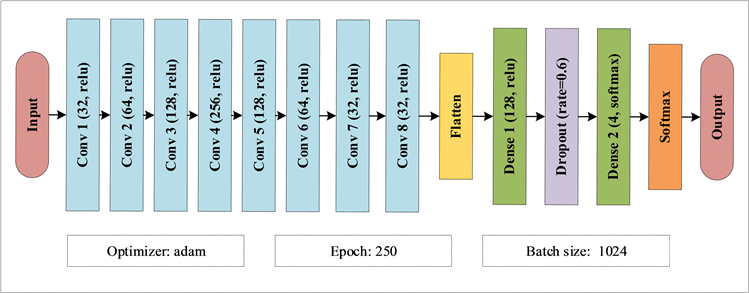Figure 5. Structure CNN model and parameters

2) 实验结果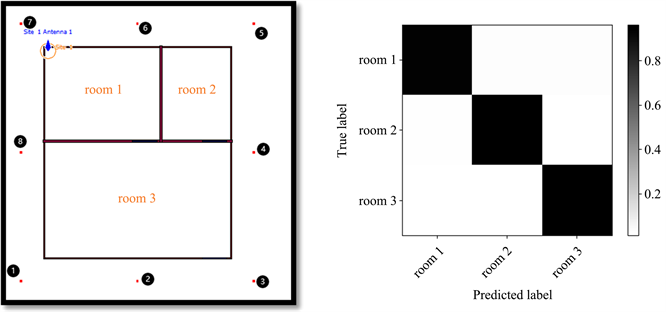Figure 6. Antenna numbering diagram and confusion matrix (8 monitoring nodes)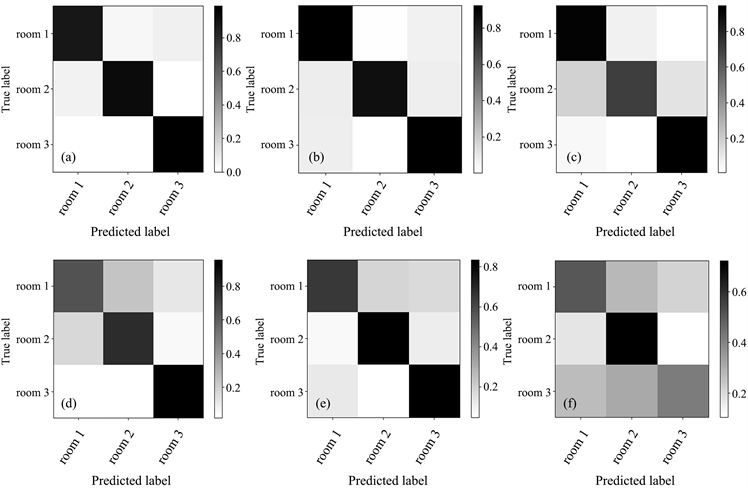Figure 7. The confusion matrix for different number of receiving points. (a) N = 7; (b) N = 6; (c) N = 5; (d) N = 4; (e) N = 3; (f) N = 2Table 1. Comparison of location recognition accuracy of CNN, KNN and SVM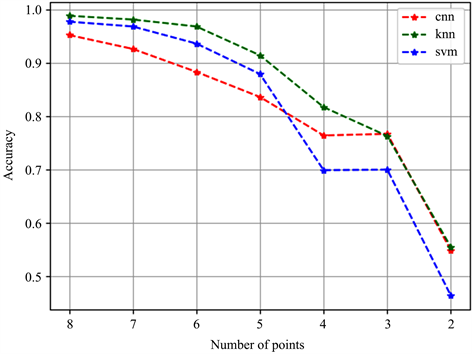Figure 8. Comparison of location recognition accuracy of three algorithms

5. 结论

  边疆无线电编委会. 边境区域无线电管理技术设施建设及投资需求研究[M]. 云南: 云南大学出版社, 2015.  中央网信办. 无线网络安全面临六大威胁[EB/OL]. http://www.cac.gov.cn/2014-03/19/c_126397441.htm  林兴峰. 企业无线网络技术安全性问题探究[J]. 电子产品可靠性与环境试验, 2020, 38(S2): 84-88.  张六, 曾正, 陈俊昌, 杨晶晶, 黄铭. 基于信道状态信息的室内人数统计方法[J]. 计算机工程与设计, 2020, 41(4): 1125-1132.  Belal, K., Hong, C. and Yasamin, M. (2021) Multiple People Identification Through Walls, Using Off-The-Shelf WiFi. IEEE Internet of Things Journal, 8, 6963-6974.  海凛, 王正斌, 程勇, 刘芫健. 基于 WinProp 仿真软件的《无线通信网络规划与优化》课程改革研究[J]. 教育现代化, 2019(74): 111-113.  Python机器学习库[DB/OL]. https://scikit-learn.org/stable/  邱锡鹏. 神经网络与深度学习[M]. 北京: 机械工业出版社, 2020.  Keras深度学习[DB/OL]. https://keras.io/about/.